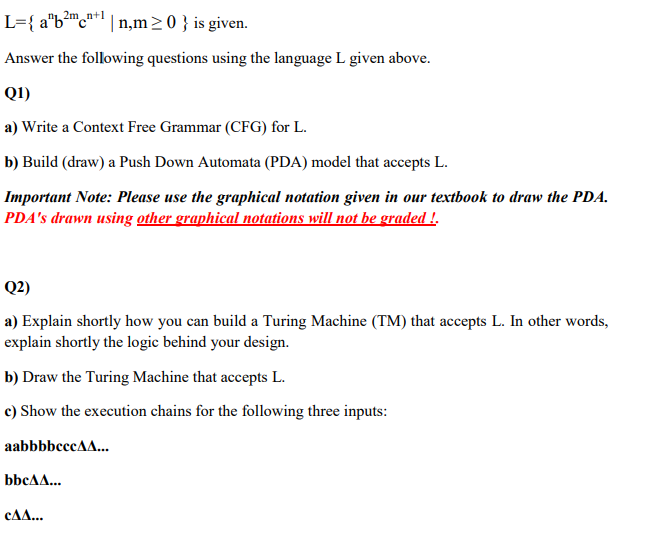Home / Expert Answers / Computer Science / mathrm-l-left-mathrm-a-mathrm-n-mathrm-b-2-mathrm-m-math-pa409

# (Solved): $$\mathrm{L}=\left\{\mathrm{a}^{\mathrm{n}} \mathrm{b}^{2 \mathrm{~m}} \math ...??????? \( \mathrm{L}=\left\{\mathrm{a}^{\mathrm{n}} \mathrm{b}^{2 \mathrm{~m}} \mathrm{c}^{\mathrm{n}+1} \mid \mathrm{n}, \mathrm{m} \geq 0\right\}$$ is given. Answer the following questions using the language L given above. Q1) a) Write a Context Free Grammar (CFG) for L. b) Build (draw) a Push Down Automata (PDA) model that accepts L. Important Note: Please use the graphical notation given in our textbook to draw the PDA. PDA's drawn using other graphical notations will not be graded!. Q2) a) Explain shortly how you can build a Turing Machine (TM) that accepts L. In other words, explain shortly the logic behind your design. b) Draw the Turing Machine that accepts L. c) Show the execution chains for the following three inputs: aabbbbcec $$\Delta \mathbf{\Delta} \ldots$$ $$\mathbf{c \Delta \Delta} \mathbf{x}$$

We have an Answer from Expert